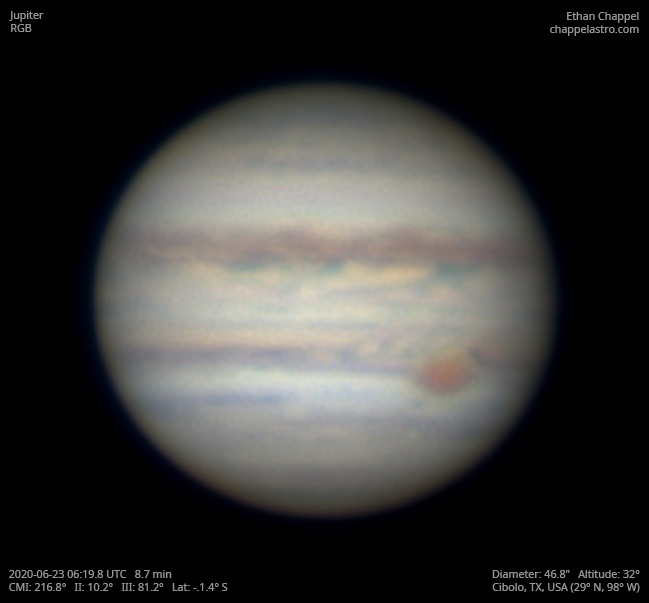# Jupiter 2020-06-23 06:19 UTC

CM1: 216.80°

CM2: 10.20°

CM3: 81.20°

CLat: -1.40°

Description

I was surprised to see a clear sky, so I started setting up. However, clouds rolled in just as I was ready, so I captured what I could between the gaps.

Notes:

• The SEBn is pale.
• Oval BA is at the central meridian.

Equipment

ZWO ASI224MC

Tele Vue 2.5x Powermate

Celestron EdgeHD 8

Logs
```FireCapture v2.6  Settings
------------------------------------
Observer=Ethan Chappel
Location=Cibolo
Scope=Celestron C14 EdgeHD
Camera=ZWO ASI224MC
Filter=CH4
Profile=Jupiter
Diameter=46.76"
Magnitude=-2.69
CMI=214.4° CMII=7.8° CMIII=78.8°  (during mid of capture)
FocalLength=4800mm (F/13)
Resolution=0.16"
Filename=2020-06-23-0616_0-EC-CH4-Jup.ser
Date=2020_06_23
Start=06_14_52.482
Mid=06_16_04.539
End=06_17_16.596
Start(UT)=06_14_52.482
Mid(UT)=06_16_04.539
End(UT)=06_17_16.596
Duration=144.114s
Date_format=yyyy_MM_dd
Time_format=HH_mm_ss
LT=UT -6h
Frames captured=2339
File type=SER
Binning=no
ROI=468x390
ROI(Offset)=0x0
FPS (avg.)=16
Shutter=46.94ms
Gain=259 (43%)
AutoGain=off
HighSpeed=off
WBlue=95 (off)
WRed=52 (off)
HardwareBin=off
AutoHisto=75 (off)
Gamma=50
SoftwareGain=10 (off)
USBTraffic=100 (off)
AutoExposure=off
Brightness=1 (off)
FPS=100 (off)
Histogramm(min)=0
Histogramm(max)=72
Histogramm=28%
Noise(avg.deviation)=0.34
Limit=none
Sensor temperature=32.0°C
Focuser position=32010
FireCapture v2.6  Settings
------------------------------------
Observer=Ethan Chappel
Location=Cibolo
Scope=Celestron C14 EdgeHD
Camera=ZWO ASI224MC
Filter=CH4
Profile=Jupiter
Diameter=46.76"
Magnitude=-2.69
CMI=218.4° CMII=11.8° CMIII=82.8°  (during mid of capture)
FocalLength=4900mm (F/13)
Resolution=0.16"
Filename=2020-06-23-0622_7-EC-CH4-Jup.ser
Date=2020_06_23
Start=06_22_26.014
Mid=06_22_42.923
End=06_22_59.832
Start(UT)=06_22_26.014
Mid(UT)=06_22_42.923
End(UT)=06_22_59.832
Duration=33.818s
Date_format=yyyy_MM_dd
Time_format=HH_mm_ss
LT=UT -6h
Frames captured=600
File type=SER
Binning=no
ROI=468x390
ROI(Offset)=0x0
FPS (avg.)=17
Shutter=46.94ms
Gain=302 (50%)
AutoGain=off
HighSpeed=off
WBlue=95 (off)
WRed=52 (off)
HardwareBin=off
AutoHisto=75 (off)
Gamma=50
SoftwareGain=10 (off)
USBTraffic=100 (off)
AutoExposure=off
Brightness=1 (off)
FPS=100 (off)
Histogramm(min)=0
Histogramm(max)=157
Histogramm=61%
Noise(avg.deviation)=0.74
Limit=180 Seconds
Sensor temperature=32.2°C
Focuser position=32010
FireCapture v2.6  Settings
------------------------------------
Observer=Ethan Chappel
Location=Cibolo
Scope=Celestron C14 EdgeHD
Camera=ZWO ASI224MC
Filter=CH4
Profile=Jupiter
Diameter=46.76"
Magnitude=-2.69
CMI=217.3° CMII=10.7° CMIII=81.7°  (during mid of capture)
FocalLength=4900mm (F/13)
Resolution=0.16"
Filename=2020-06-23-0620_8-EC-CH4-Jup.ser
Date=2020_06_23
Start=06_19_21.917
Mid=06_20_51.922
End=06_22_21.927
Start(UT)=06_19_21.917
Mid(UT)=06_20_51.922
End(UT)=06_22_21.927
Duration=180.010s
Date_format=yyyy_MM_dd
Time_format=HH_mm_ss
LT=UT -6h
Frames captured=3089
File type=SER
Binning=no
ROI=468x390
ROI(Offset)=0x0
FPS (avg.)=17
Shutter=46.94ms
Gain=293 (48%)
AutoGain=off
HighSpeed=off
WBlue=95 (off)
WRed=52 (off)
HardwareBin=off
AutoHisto=75 (off)
Gamma=50
SoftwareGain=10 (off)
USBTraffic=100 (off)
AutoExposure=off
Brightness=1 (off)
FPS=100 (off)
Histogramm(min)=0
Histogramm(max)=158
Histogramm=61%
Noise(avg.deviation)=0.68
Limit=180 Seconds
Sensor temperature=32.1°C
Focuser position=32010
```